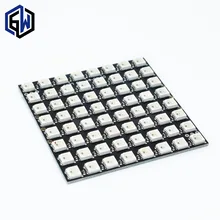Home Code Neomatrix scrolling text example on an Arduino

# Neomatrix scrolling text example on an Arduino

In a previous example we used Neopixel libraries and had a couple of code examples. Now we look at a couple of different libraries, the example built in scrolls text on the screenAliExpress.com Product – WS2812 LED 5050 RGB 8×8 64 LED Matrix for Arduino

### Code Example

You need the Adafruit GFX library, Adafruit Neopixel and Adafruit Neomatrix libraries – all of these are installable via the library manager

 Source code```// Adafruit_NeoMatrix example for single NeoPixel Shield.

#ifndef PSTR
#define PSTR // Make Arduino Due happy
#endif

#define PIN 3

// MATRIX DECLARATION:
// Parameter 1 = width of NeoPixel matrix
// Parameter 2 = height of matrix
// Parameter 3 = pin number (most are valid)
// Parameter 4 = matrix layout flags, add together as needed:
//   NEO_MATRIX_TOP, NEO_MATRIX_BOTTOM, NEO_MATRIX_LEFT, NEO_MATRIX_RIGHT:
//     Position of the FIRST LED in the matrix; pick two, e.g.
//     NEO_MATRIX_TOP + NEO_MATRIX_LEFT for the top-left corner.
//   NEO_MATRIX_ROWS, NEO_MATRIX_COLUMNS: LEDs are arranged in horizontal
//     rows or in vertical columns, respectively; pick one or the other.
//   NEO_MATRIX_PROGRESSIVE, NEO_MATRIX_ZIGZAG: all rows/columns proceed
//     in the same order, or alternate lines reverse direction; pick one.
//   See example below for these values in action.
// Parameter 5 = pixel type flags, add together as needed:
//   NEO_KHZ800  800 KHz bitstream (most NeoPixel products w/WS2812 LEDs)
//   NEO_KHZ400  400 KHz (classic 'v1' (not v2) FLORA pixels, WS2811 drivers)
//   NEO_GRB     Pixels are wired for GRB bitstream (most NeoPixel products)
//   NEO_RGB     Pixels are wired for RGB bitstream (v1 FLORA pixels, not v2)

// Example for NeoPixel Shield.  In this application we'd like to use it
// as a 5x8 tall matrix, with the USB port positioned at the top of the
// Arduino.  When held that way, the first pixel is at the top right, and
// lines are arranged in columns, progressive order.  The shield uses
// 800 KHz (v2) pixels that expect GRB color data.
NEO_MATRIX_TOP     + NEO_MATRIX_RIGHT +
NEO_MATRIX_COLUMNS + NEO_MATRIX_PROGRESSIVE,
NEO_GRB            + NEO_KHZ800);

const uint16_t colors[] = {
matrix.Color(255, 0, 0), matrix.Color(0, 255, 0), matrix.Color(0, 0, 255) };

void setup()
{
matrix.begin();
matrix.setTextWrap(false);
matrix.setBrightness(5);
matrix.setTextColor(colors);
}

int x    = matrix.width();
int pass = 0;

void loop()
{
matrix.fillScreen(0);
matrix.setCursor(x, 0);
matrix.print(F("Arduino"));
if(--x < -36) {
x = matrix.width();
if(++pass >= 3) pass = 0;
matrix.setTextColor(colors[pass]);
}
matrix.show();
delay(100);
}```

All going well Arduino will scroll on the Neomatrix display